# The World of Clean Clutters, I: Ideal Clutters

You may have heard of Paul Seymour’s f-Flowing Conjecture on binary matroids, perhaps as alluded to in these detailed posts (I, II, III) by Dillon Mayhew, or in connection with weakly bipartite graphs, or directly from the source. If you haven’t heard of it, or have but don’t remember much, or better yet, know it well but need a new lens to view the problem, it’s your lucky day! I’m here to tell you all about it.

For you to fully appreciate the conjecture though, I’ll have to put it in the proper context. That’ll require me to take you outside your comfort zone of matroids to the larger world of clutters, where I’ll be able to talk about ideal clutters and binary clutters. I’ll then be able to present the f-Flowing Conjecture, the latest progress made towards resolving it, as well as the tools that have made that possible.

But I won’t stop there. I’ll talk about an adjacent, intriguing class of clutters, those without an intersecting clutter as a minor, and then move my way up to the all-encompassing class of clean clutters (an oxymoron, I know!): a minor-closed class whose excluded minors come from degenerate projective planes and odd holes.

This series of posts is meant to provide a non-technical survey of the classes displayed below. I shall provide a historical account of each class, hopefully making it clear why the class is interesting, and then provide just one exciting conjecture to stimulate further research on the class.

This post focuses on the class of Ideal Clutters.

1.

Clutters form the framework for my post. I’m grateful that Dillon has already laid the basics in these posts: I, II, III, but let me recall a few definitions, as well as make some new ones; the careful reader will notice that my notation is slightly but harmlessly different from Dillon’s.

Let E be a finite set of elements, and let $\mathcal{C}$ be a family of subsets of E called members. $\mathcal{C}$ is a clutter over ground set E if no member contains another one.

A cover of $\mathcal{C}$ is a subset of E that intersects every member. Every superset of a cover is another cover, so not all covers are interesting from a clutter theoretic perspective, thereby leading us to the forthcoming adjective. A cover is minimal if it does not contain another cover.

By design, the family of minimal covers of $\mathcal{C}$ forms another clutter over the same ground set. This clutter is called the blocker of $\mathcal{C}$, and is denoted $b(\mathcal{C})$. Dillon has already proved the basic fact that the blocking relation is an involution, that is, $b(b(\mathcal{C}))=\mathcal{C}$.

Our choice of terminology for “clutter” and “blocker” follows the convention set forth by Jack Edmonds and Ray Fulkerson in this 1970 paper. The origin of the blocking relation, however, dates earlier to the theory of games, wherein the members of $\mathcal{C}$ represent the minimal winning coalitions, while the blocker takes an adversarial position, collecting all the minimal hitting sets, meant to tear down every winning coalition. For instance, these important 1958 and 1965 papers lay some of the foundational concepts in the study of clutters, predating the Edmonds-Fulkerson paper.

Given disjoint subsets $I,J\subseteq E$, $\mathcal{C}\setminus I/J$ denotes the clutter over ground set $E-(I\cup J)$ whose members are the inclusionwise minimal sets of $\{C-J: C\in \mathcal{C}, C\cap I=\emptyset\}$. This clutter is called the minor of $\mathcal{C}$ obtained after deleting $I$ and contracting $J$. This notion was coined in 1971 by Ray Fulkerson

You might find the notions of the blocker and clutter minors reminiscent of matroid duality and matroid minors, in which case you’d be happily surprised that the former two notions may in fact be viewed as extensions of the latter two.

For example, let $M$ be a matroid over ground set $E$, and take an element $f\in E$. Denote by $\mathrm{port}(M,f)$ the clutter over ground set $E-\{f\}$ whose members are $$\{C-\{f\} : C \text{ is a circuit of M containing f}\}.$$ Seymour refers to this clutter as a port of $M$. He has shown that,

• $b(\mathrm{port}(M,f)) = \mathrm{port}(M^\star,f)$ (Dillon proved this in his first post on clutters),
• $\mathrm{port}(M,f)\setminus I/J = \mathrm{port}(M\setminus I/J,f)$ for disjoint $I,J\subseteq E-\{f\}$.

Thus, the two clutter notions are indeed extensions of matroid duality and matroid minors. One may have reached this conclusion alternatively by taking the clutter of bases, or circuits, of a matroid.

2.

Now that we’re done with the basics of Clutter Theory, we can move on to the main topic of this post: Ideal Clutters.

$\mathcal{C}$ is an ideal clutter if any of the following equivalent conditions are satisfied:

1. Every vertex of the polyhedron $Q(\mathcal{C}):=\{x\in \mathbb{R}^E_+: x(C)\geq 1 ~ \forall C\in \mathcal{C}\}$ is integral.
2. For all cost vectors $c\in \mathbb{R}^E$, the linear program $$\text{minimize } c^\top x \text{ subject to } x\in Q(\mathcal{C})$$ if finite has an integral optimal solution.
3. For all lengths $\ell\in \mathbb{R}^E_+$ and widths $w\in \mathbb{R}^E_+$, the width-length inequality holds: $$\min\{\ell(C):C\in \mathcal{C}\}\cdot \min\{w(B):B\in b(\mathcal{C})\}\leq \ell^\top w.$$

Here, $x(C)$ is short-hand notation for $\sum_{e\in C}x_e$.

The term ideal was coined in 1994 by Gérard Cornuéjols and Beth Novick, who used #1 as the main definition. This concept has been dubbed in the literature as the weak max-flow min-cut property, the max-flow min-cut property, the width-length property, or even the Fulkersonian property, all of which only speaks to the rich and tangled history of the concept, hinted at by the following table, displaying various classes of ideal clutters in the literature.

In 1963 Alfred Lehman, inspired by Moore and Shannon’s landmark work on unreliable communication networks, studied the width-length inequality, condition #3, and proved it equivalent to #1, and also to what he called the max-flow min-cut equality, #2. In case you’re wondering what the terminology refers to, apply Strong Duality to the LP in #2.

The characterization #3 of idealness is particularly interesting due to its consequence that

the blocker of an ideal clutter is also ideal.

The blocking relation between clutters was later extended to polyhedra by Fulkerson, as documented in this RAND report. In the 1968 memorandum, he develops a geometric view of the blocking relation and gives another proof that idealness is closed under the blocking relation. Sadly, some authors fail to cite Lehman for this fact and only cite Fulkerson’s 1971 published version of the report, even though Ray himself cites Lehman for the fact. Understandably, Lehman did not put his 1963 memorandum in print until 1979!

3.

Not only is idealness closed under the blocking relation, but

every minor of an ideal clutter is also ideal.

I leave the proof of this as an exercise for the reader; the only thing I’ll say is that there are three proofs, one corresponding to each characterization of idealness.

Given the fact above, a natural question arises:

What are the excluded minors for the class of ideal clutters?

A clutter is minimally non-ideal (mni) if it is non-ideal but every proper minor is ideal. Observe that a clutter is ideal if, and only if, it has no mni minor. In particular, the excluded minors for the class of ideal clutters are precisely the mni clutters.

Note that as idealness is closed under the blocking relation, and deletion/contraction corresponds to contraction/deletion in the blocker, the blocker of every mni clutter is another mni clutter.

In 1963, Lehman found four classes of mni minors, three of which formed infinite classes:

Lehman seems to have been discouraged by the multiplicity of the excluded minors:

“Whether or not this list is complete, the multiplicity of minimal matrices seems to preclude their usefulness as a W-L matrix characterization. (Cf. author’s foreword.)”

In the foreword, which was added 17 years later in 1979 when the paper was finally published, he writes:

“More is known about minimal non-W-L matrices. U. Peled has found several additional classes of matrices which are also classically known in other contexts.”

I’m not sure what additional classes Uri Peled had found at the time but to this day, there are over 1500 small examples of mni clutters: See the paper by Cornuejols and Novick, as well as this paper by Lütolf and Margot. A new infinite class of mni clutters was also found 9 years ago (and don’t forget, the blocking clutters form another infinite class).

Lehman continues in the foreword:

“A consequence of a recent matrix theorem is apparently that the degenerate projective planes [i.e. the deltas] are the only minimal matrices requiring unequal weights.”

More specifically, the consequence of the “matrix theorem” states that for a minimally non-ideal clutter $\mathcal{C}$ different from $\Delta_4$, $\Delta_5$,…,, the width-length inequality is violated for $w=\ell={\bf 1}.$ Whereas for the mni clutters $\Delta_4$, $\Delta_5$,…, the width-length inequality is satisfied for $w=\ell={\bf 1}$.

The foreword above is the humblest of hints to one of the deepest results on ideal clutters, a result that dusted in Lehman’s desk drawer for over 10 years, until it finally appeared in 1990 at the request of Bill Cook and Paul Seymour in the first issue of DIMACS Series. A year later, Lehman won a Fulkerson prize for this “matrix theorem”. If you’re curious about the statement of this theorem, I urge you to read Chapter 9 of my notes, and in particular, Theorem 9.12.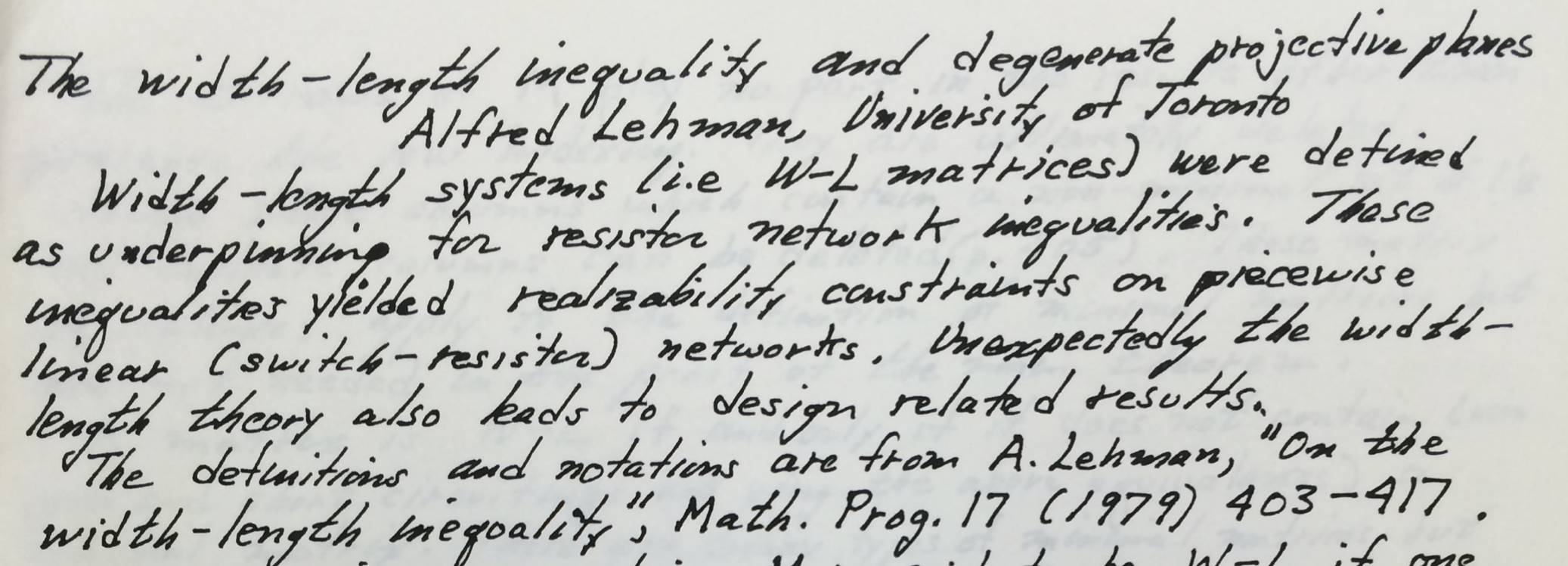A snapshot of Lehman’s handwritten manuscript on the special role of the deltas among minimally non-ideal clutters.

If you, like Lehman himself, are skeptical about the usefulness of his matrix theorem, the next post is bound to change your mind.

4.

Before I conclude with ideal clutters, let me leave you with a conjecture on idealness that Gérard Cornuéjols, Tony Huynh, Dabeen Lee, and I made in a paper whose extended abstract appeared in the proceedings of IPCO 2020, held online this past summer at the London School of Economics.

Suppose $\mathcal{C}$ has no member of cardinality one. A proper colouring of $\mathcal{C}$ is an assignment of colours to the elements so that there is no monochromatic member, that is, it consists of an assignment of an integer $\phi(e)$ to every element $e$ such that $\{\phi(e):e\in C\}$ has cardinality at least two. The chromatic number of $\mathcal{C}$ is the minimum number of colours used in a proper colouring.

Conjecture. There exists an integer $k\geq 4$ such that every ideal clutter without a member of cardinality one has chromatic number at most $k$.

Why $k$ should be at least four, and part of the origins of the conjecture, are explained in the paper. I hope the abrupt appearance of the conjecture is made up for by its utter simplicity.

Thank you for sticking around long enough to read this sentence. If you have any questions, feel free to ask them in the comment section below.

Ahmad Abdi, London School of Economics

# Matroids over Partial Hyperstructures

Guest Post by Matt Baker.

In this post I’d like to explain a new generalization of matroids developed in this recent paper with Nathan Bowler (referred to henceforth as [BB]). We show that by working over certain algebraic structures which we call tracts, linear subspaces, matroids, valuated matroids, oriented matroids, and matroids over partial fields all become special cases of a single general concept. Actually, there are at least two natural notions of matroids over tracts, which we call weak and strong matroids, but in many cases of interest (such as all the ones mentioned above) the two notions coincide.

Two important special cases of tracts are hyperfields and partial fields. We assume familiarity with the theory of partial fields (see for example this post and its sequels by Stefan van Zwam).

Hyperfields

Roughly speaking, a hyperfield is an algebraic structure satisfying similar axioms to a field, but where addition is allowed to be multi-valued. Like fields, hyperfields come equipped with an additive identity element called zero and a negation map $x \mapsto -x$. However, one does not require that the hypersum of $x$ and $-x$ is zero, only that zero is contained in the hypersum. Before giving a precise axiomatic definition, let us give some motivating examples.

1. (Fields) Any field $K$ is in particular a hyperfield.
2. (The Krasner hyperfield) The Krasner hyperfield ${\mathbb K}$ records the arithmetic of “zero” versus “nonzero”. Specifically, define ${\mathbb K} = \{ 0,1 \}$ together with the binary operation $0 \odot 0 = 0, 0 \odot 1 = 0, 1 \odot 1 = 1$ and the binary hyperoperation $0 \boxplus 0 = \{ 0 \}, 0 \boxplus 1 = \{ 1 \}, 1 \boxplus 1 = \{ 0,1 \}$. If we think of $1$ as representing “nonzero”, the hyperaddition rules just say that zero plus zero is zero and zero plus something nonzero is always nonzero, but the sum of two things which are nonzero could be either zero or nonzero. The negation operator is the identity map, since negative zero is zero and the negative of something nonzero is again nonzero.
3. (The hyperfield of signs) The hyperfield of signs ${\mathbb S}$ records the arithmetic of “zero”, “positive”, and “negative”, represented by the symbols $0, 1, -1$, respectively. The product $x \odot y$ is defined in the obvious way for $x,y \in {\mathbb S} := \{ 0, 1, -1 \}$. Addition is also defined in the “obvious” way except we have $1 \boxplus -1 = \{ 0, 1, -1 \}$ since the sum of a positive number and a negative number could be either zero, positive, or negative. The negation operator takes $0$ to itself and interchanges $1$ and $-1$.
4. (The tropical hyperfield) The tropical hyperfield ${\mathbb T}$ records the arithmetic of valuations. More precisely, if $v : K \to {\mathbb T} := {\mathbb R} \cup \{ \infty \}$ is the valuation map on a valued field $K$ with value group ${\mathbb R}$, the hypersum $a \boxplus b$ consists of all possible values of $v(\alpha+\beta)$ where $\alpha,\beta$ are elements of $K$ with $v(\alpha)=a$ and $v(\beta)=b$. (Note that the axioms for a valuation tell us that $v(\alpha \cdot\beta) = a + b$.) Concretely, this means that we should define $a \odot b := a + b$ (with the evident conventions when one of $a,b$ equals $\infty$), and we define $a \boxplus b := {\rm min}(a,b)$ if $a \neq b$ and $a \boxplus a := \{ z \in {\mathbb T} \; : \; z \geq a \}$. The multiplicative identity element is $0$ and the additive identity element is $\infty$. The negation operator is the identity map, since the unique value of $b$ such that $\infty \in a \boxplus b$ is $b = a$.

The above examples all have an additional property not shared by all hyperfields: they are doubly distributive (see below for the definition). Here are two examples of hyperfields which are not doubly distributive:

5. (The triangle hyperfield) Let ${\mathbb V}$ be the set ${\mathbb R}_{\geq 0}$ of nonnegative real numbers with the usual multiplication and the hyperaddition rule $a \boxplus b := \{ c \in {\mathbb R}_{\geq 0} \; : \; |a-b| \leq c \leq a+b \}.$ In other words, $a \boxplus b$ is the set of all real numbers $c$ such that there exists a (possibly degenerate) Euclidean triangle with side lengths $a, b, c$. Then ${\mathbb V}$ is a hyperfield.

6. (The phase hyperfield) The phase hyperfield ${\mathbb P}$ records the arithmetic of phases of complex numbers. If $\pi : {\mathbb C} \to {\mathbb P} := S^1 \cup \{ 0 \}$ is the map taking 0 to 0 and $z \in {\mathbb C}^*$ to $z/|z|$, and if $a,b \in {\mathbb P}$, the hypersum $a \boxplus b$ consists of all possible values of $\pi(\alpha + \beta)$ where $\alpha,\beta$ are elements of ${\mathbb C}$ with $\pi(\alpha)=a$ and $\pi(\beta)=b$. More precisely, multiplication in ${\mathbb P}$ is defined as usual, and the hyperaddition law is defined for $x,y \neq 0$ by setting $x \boxplus -x := \{ 0, x, -x \}$ and $x \boxplus y := \{ \frac{\alpha x + \beta y}{\| \alpha x + \beta y \|} \; | \; \alpha, \beta \in {\mathbb R}_{>0} \}$ otherwise.
Motivated by Proposition 1, if $F$ is a tract we define a strong $F$-matroid (or strong matroid over $F$) of rank $r$ on $E$ to be a projective equivalence class of Grassmann-Plücker functions $\varphi : E^r \to F$. Thus strong matroids over fields are the same as linear subspaces, and strong matroids over the Krasner hyperfield are the same as matroids in the usual sense. (By a matroid over a partial hyperfield $F$, we mean a matroid over the corresponding tract.) One can also show that strong matroids over a partial field $P$ are the same as matroids representable over $P$ in the usual sense.

Definition of hyperrings and hyperfields

A hyperoperation on a set $S$ is a map $\boxplus$ from $S \times S$ to the collection of non-empty subsets of $S$.

If $A,B$ are non-empty subsets of $S$, we define $A \boxplus B := \bigcup_{a \in A, b \in B} (a \boxplus b)$ and we say that $\boxplus$ is associative if $a \boxplus (b \boxplus c) = (a \boxplus b) \boxplus c$ for all $a,b,c \in S$.

A (commutative) hyperring is a set $R$ together with:

1. A commutative and associative binary operation $\odot$
2. A commutative and associative binary hyperoperation $\boxplus$
3. Distinguished elements $0,1 \in R$

such that:

1. $(R, \odot, 1)$ is a commutative monoid.
2. $0 \odot x = x \odot 0 = 0$ and $0 \boxplus x = x \boxplus 0 = \{ x \}$ for all $x \in R.$
3. For every $x \in R$ there is a unique element of $R$ (denoted $-x$) such that $0 \in x\boxplus -x.$
4. $a \odot (x \boxplus y) = (a \odot x) \boxplus (a \odot y)$ for all $a,x,y \in R.$

A hyperring $R$ is called a hyperdomain if $xy=0$ in $R$ implies that $x=0$ or $y=0$.

A hyperring $R$ is called doubly distributive if it satisfies $(a \boxplus b)\odot (c \boxplus d) = (a\odot c) \boxplus (a \odot d) \boxplus (b \odot c) \boxplus (b\odot d)$ for all $a,b,c,d \in R$.

A hyperring $F$ is called a hyperfield if $0 \neq 1$ and every non-zero element of $F$ has a multiplicative inverse.

Partial hyperfields

A partial hyperfield is a pair $(G,R)$, where $G$ is a subgroup of the group of units of a hyperdomain $R$ such that $-1 \in R$ and $G$ generates $R$ as a hyperring.

We set $P := G \cup \{ 0 \}$, and denote the partial hyperfield $(G,R)$ simply by $P$ when no confusion is likely to arise.

Partial hyperfields generalize both hyperfields and partial fields in a natural way.  When $R$ is a ring, $P$ is just a partial field, and when $G = R \backslash \{ 0 \}$ is a group, $P$ is just a hyperfield.

A partial hyperfield is called doubly distributive if the ambient hyperring $R$ is doubly distributive.

Tracts

Partial hyperfields are special cases of still more general objects called tracts, which appear to be a natural setting for matroid theory.

We define a tract to be an abelian group $G$ (written multiplicatively), together with a subset $N_G$ of the group semiring ${\mathbb N}[G]$ satisfying:

(T0) The zero element of ${\mathbb N}[G]$ belongs to $N_G$.
(T1) The identity element 1 of $G$ is not in $N_G$.
(T2) There is a unique element $\epsilon$ of $G$ with $1 + \epsilon \in N_G$.
(T3) $N_G$ is closed under the natural action of $G$ on ${\mathbb N}[G]$.

We set $F := G \cup \{ 0 \} \subset {\mathbb N}[G]$, and refer to the tract $(G,N_G)$ simply as $F$ when no confusion is likely to arise.  We will also sometimes write $F^\times$ instead of $G$, and $-1$ instead of $\epsilon$.

One thinks of $N_G$ as those linear combinations of elements of $G$ which “sum to zero” (the $N$ in $N_G$ stands for “null”).

Tracts from partial hyperfields

We can associate a tract to a partial hyperfield $(G,R)$ by declaring that a formal sum $\sum a_i g_i \in {\mathbb N}[G]$ belongs to $N_G$ if and only if $0 \in \boxplus a_i g_i$ in $R$.

We note, for the experts, that one can associate a tract to any fuzzy ring in the sense of Dress and Dress-Wenzel, and that if $P$ is a doubly distributive partial hyperfield there is an associated fuzzy ring whose realization as a tract coincides with the realization of $P$ itself as a tract.

Grassmann-Plücker functions over tracts

Now let $F$ be a tract.  A function $\varphi : E^r \to F$ is called a Grassmann-Plücker function if it is nonzero, alternating (meaning that $\varphi(x_1,\ldots,x_i, \ldots, x_j, \ldots, x_r)= -\varphi(x_1,\ldots,x_j, \ldots, x_i, \ldots, x_r)$ and $\varphi(x_1,\ldots, x_r) = 0$ if $x_i = x_j$ for some $i \neq j$), and it satisfies the following generalization of the classical Grassmann-Plücker relations:

(GP) For any two subsets $X := \{ x_1,\ldots,x_{r+1} \}$ and $Y := \{ y_1,\ldots,y_{r-1} \}$ of $E$,
$\sum_{k=1}^{r+1} (-1)^k \varphi(x_1,x_2,\ldots,\hat{x}_k,\ldots,x_{r+1}) \cdot \varphi(x_k,y_1,\ldots,y_{r-1}) \in N_G.$

If $F=K$ is a field then a projective equivalence class of Grassmann-Plücker functions $\varphi : E^r \to K$ is the same thing as an $r$-dimensional subspace of $K^m$.  This is essentially just the assertion that the usual Grassmannian variety is cut out by the Plücker equations.

On the other hand:

Proposition 1: If $F = {\mathbb K}$ is the Krasner hyperfield, a projective equivalence class of Grassmann-Plücker functions $\varphi : E^r \to {\mathbb K}$ is the same thing as a rank $r$ matroid on $E$.

Indeed, if $\varphi : E^r \to {\mathbb K}$ is a nonzero alternating function and ${\mathbf B}_\varphi$ denotes the set of $r$-element subsets $\{ e_1,\ldots, e_r \}$ of $E$ such that $\varphi(e_1,\ldots,e_r) \neq 0$, it is easy to see that ${\mathbf B} := {\mathbf B}_\varphi$ satisfies the Grassmann-Plücker relations (GP) if and only if

(BE) Given $B,B’ \in {\mathbf B}$ and $b \in B \backslash B’$, there exists $b’ \in B’ \backslash B$ such that both $(B \cup \{ b’ \}) \backslash \{ b \}$ and $(B’ \cup \{ b \}) \backslash \{ b’ \}$ are in ${\mathbf B}$.

But condition (BE) is well-known to be equivalent to the usual Basis Exchange property for matroids!  In other words, ${\mathbf B}_\varphi$ is the set of bases of a rank $r$ matroid $M_{\varphi}$ on $E$.  Conversely, given such a matroid $M$, we can define $\varphi_M : E^r \to {\mathbb K}$ by setting $\varphi_M(e_1,\ldots,e_r) = 1$ if $\{ e_1,\ldots,e_r \}$ is a basis of $M$ and $\varphi_M(e_1,\ldots,e_r) = 0$ if not.  By the exchange property (BE), the function $\varphi_M(e_1,\ldots,e_r)$ will satisfy (GP).

Motivated by Proposition 1, if $F$ is a tract we define a strong $F$-matroid (or strong matroid over $F$) of rank $r$ on $E$ to be a projective equivalence class of Grassmann-Plücker functions $\varphi : E^r \to F$.  Thus strong matroids over fields are the same as linear subspaces, and strong matroids over the Krasner hyperfield are the same as matroids in the usual sense.   (By a matroid over a partial hyperfield $F$, we mean a matroid over the corresponding tract.)  One can also show that strong matroids over a partial field $P$ are the same as matroids representable over $P$ in the usual sense.

We have the following additional interesting examples of (strong) matroids over tracts:

Proposition 2: If $F = {\mathbb S}$ is the hyperfield of signs, a matroid over ${\mathbb S}$ is the same thing as an oriented matroid.

Proposition 3: If $F = {\mathbb T}$ is the tropical hyperfield, a matroid over ${\mathbb T}$ is the same thing as a valuated matroid.

Proposition 4: If $F = {\mathbb U}_0$ is the regular partial field $(\{ \pm1 \}, {\mathbb Z})$, a matroid over ${\mathbb U}_0$ is the same thing as a regular matroid.

Weak matroids over tracts

It is also of interest to consider objects which satisfy a weaker version of (GP), where we consider only the three-term Grassmann-Plücker relations. Specifically, a weak $F$-matroid is a projective equivalence class of nonzero alternating functions $\varphi : E^r \to F$ such that (a) ${\mathbf B}_\varphi$ is the set of bases for a (classical) matroid on $E$, and (b) $\varphi$ satisfies (GP) for all $X,Y$ with $|X \backslash Y| = 3$.

It will turn out that in Propositions 1-4 above, strong and weak $F$-matroids are the same.

Circuit axioms for matroids over tracts

Let ${\mathcal C}$ be a collection of pairwise incomparable nonempty subsets of $E$. We say that $C_1,C_2 \in {\mathcal C}$ form a modular pair in ${\mathcal C}$ if $C_1 \neq C_2$ and $C_1 \cup C_2$ does not properly contain a union of two distinct elements of ${\mathcal C}$.

If $F$ is a tract and $X \in F^m$, we write $\underline{X}$ for the support of $X$, i.e., the set of $i \in \{ 1,\ldots,m \}$ such that $X_i \neq 0$. If ${\mathcal C} \subset F^m$ and $X,Y \in {\mathcal C}$, we say that $X,Y$ are a modular pair in ${\mathcal C}$ if $\underline{X},\underline{Y}$ are a modular pair in $\underline{\mathcal C} := \{ \underline{X} \; : \; X \in {\mathcal C} \}.$

Theorem 1: Let $F$ be a tract and let $E = \{ 1,\ldots,m \}$. There is a natural bijection between weak $F$-matroids on $E$ and collections ${\mathcal C} \subset F^m$ satisfying:

(C0) $0 \not\in {\mathcal C}$.
(C1) If $X \in {\mathcal C}$ and $\alpha \in F^\times$, then $\alpha \cdot X \in {\mathcal C}$.
(C2) [Incomparability] If $X,Y \in {\mathcal C}$ and $\underline{X} \subseteq \underline{Y}$, then there exists $\alpha \in F^\times$ such that $X = \alpha \cdot Y$.
(C3) [Modular Elimination] If $X,Y \in {\mathcal C}$ are a modular pair in ${\mathcal C}$ and $e \in E$ is such that $X_e= -Y_e \neq 0$, there exists $Z \in {\mathcal C}$ such that $Z_e=0$ and $X_f + Y_f – Z_f \in N_G$ for all $f \in E$.

We call ${\mathcal C}$ the set of $F$-circuits of the weak $F$-matroid $M$.

In [BB], there is also a stronger version of the circuit elimination axiom (C3) which gives a cryptomorphic characterization of strong $F$-matroids in terms of circuits. Let’s say that a family of atomic elements of a lattice is modular if the height of their join in the lattice is the same as the size of the family. If $\mathcal C$ is a subset of $F^m$, a modular family of elements of $\mathcal C$ is one such that the supports give a modular family of elements in the lattice of unions of supports of elements of $\mathcal C$.

Then there is a natural bijection between strong $F$-matroids on $E$ and collections ${\mathcal C} \subset F^m$ satisfying (C0),(C1),(C2), and the following stronger version of the modular elimination axiom (C3):

[Strong modular elimination] Suppose $X_1,\ldots,X_k$ and $X$ are $F$-circuits of $M$ which together form a modular family of size $k+1$ such that $\underline X \not \subseteq \bigcup_{1 \leq i \leq k} \underline X_i$, and for $1 \leq i \leq k$ let $e_i \in (X \cap X_i) \setminus \bigcup_{\substack{1 \leq j \leq k \\ j \neq i}} X_j$ be such that $X(e_i) = -X_i(e_i) \neq 0$. Then there is an $F$-circuit $Z$ such that $Z(e_i) = 0$ for $1 \leq i \leq k$ and $X_1(f) + \cdots + X_k(f) + X(f) – Z(f) \in N_G$ for every $f \in E$.

Duality

There is a duality theory for matroids over tracts which generalizes the known duality theories for matroids, oriented matroids, valuated matroids, etc., and which corresponds to orthogonal complementation for matroids over fields.

Let $F$ be a tract. The inner product of two vectors $X,Y \in F^m$ is $X \cdot Y := \sum_{i=1}^m X_i \cdot Y_i.$ We call $X$ and $Y$ orthogonal, denoted $X \perp Y$, if $X \cdot Y \in N_G$. If $S \subset F^m$, we denote by $S^\perp$ the orthogonal complement of $S$, i.e., the set of all $X \in F^m$ such that $X \perp Y$ for all $Y \in S$.

If $M$ is a (weak or strong) $F$-matroid on $E$ whose collection of circuits is denoted ${\mathcal C}$, then $\underline{M} := \{ \underline{X} \; : \; X \in {\mathcal C} \}$ is the collection of circuits of a matroid in the usual sense on $E$, which we call the underlying matroid of $M$.

Theorem 2: Let $F$ be a tract, and let $M$ be a (weak, resp. strong) $F$-matroid of rank $r$ on $E=\{ 1,\ldots,m \}$ with $F$-circuit set ${\mathcal C}$ and Grassmann-Plücker function $\varphi.$
Then there is a (weak, resp. strong) $F$-matroid $M^*$ of rank $m-r$ on $E$, called the dual $F$-matroid of $M$, with the following properties:

1. The $F$-circuits of $M^*$ are the elements of ${\mathcal C}^\perp$ of minimal non-empty support.

2. $M^*$ has the Grassmann-Plücker function $\varphi^*(x_1,\ldots,x_{m-r}) = {\rm sign}(x_1,\ldots,x_{m-r},x_1′,\ldots,x_r’) \varphi(x_1′,\ldots,x_r’),$ where $x_1′,\ldots,x_r’$ is any ordering of $E \backslash \{ x_1,\ldots,x_{m-r} \}.$

3. The underlying matroid of $M^*$ is the dual of the underlying matroid of $M$.

4. $M^{**} = M$.

The deepest part of Theorem 2 is the fact that the elements of ${\mathcal C}^\perp$ of minimal non-empty support satisfy the circuit elimination axiom (C3) (or its strong variant).

Dual pair axioms for matroids over hyperfields

One can give another cryptomorphic characterization of $F$-matroids using the notion of dual pairs. It is perhaps the simplest of all descriptions of matroids over tracts, but it presupposes that one already knows what a (usual) matroid is.

Let $M$ be a (classical) matroid on $E$. We call a subset ${\mathcal C}$ of $F^m$ an $F$-signature of $M$ if it is closed under multiplication by nonzero elements of $F$ and $\underline{\mathcal C} := \{ \underline{X} \; : \; X \in {\mathcal C} \}$ is the set of circuits of $M$.

We call $({\mathcal C},{\mathcal D})$ a dual pair of $F$-signatures of $M$ if:

(DP1) ${\mathcal C}$ is an $F$-signature of $M$.
(DP2) ${\mathcal D}$ is an $F$-signature of the dual matroid $M^*$.
(DP3) ${\mathcal C} \perp {\mathcal D}$, meaning that $X \perp Y$ for all $X \in {\mathcal C}$ and $Y \in {\mathcal D}$.

Theorem 3: Let $F$ be a tract and let $E = \{ 1,\ldots,m \}$. There is a natural bijection between strong $F$-matroids on $E$ and matroids $M$ on $E$ together with a dual pair of $F$-signatures of $M$.

An analogous theorem holds for weak $F$-matroids if we only require that (DP3) holds when $|\underline{X} \cap \underline{Y}| \leq 3$.

Vectors, perfection, and double distributivity

Given a tract $F$ and a strong $F$-matroid $M$ on $E$ with $F$-circuit set $\mathcal C$ and $F$-cocircuit set $\mathcal C^*$, a vector of $M$ is an element of $F^m$ which is orthogonal to everything in $\mathcal C^*$. Similarly a covector of $M$ is an element of $F^m$ which is orthogonal to everything in $\mathcal C$. We say that $F$ is perfect if, for any strong matroid $M$ over $F$, all vectors are orthogonal to all covectors.

Theorem 4: Let $F$ be a tract.

1. If $F$ is perfect, then the notions of weak and strong $F$-matroids coincide.

2. Every doubly distributive partial hyperfield is perfect.

As a consequence, we see that the notions of weak and strong $F$-matroids coincide for doubly distributive partial hyperfields $F$. This holds, in particular, when $F=P$ is a partial field or when $F$ is the Krasner hyperfield, the tropical hyperfield, or the hyperfield of signs.

There are examples in [BB] which show that weak and strong $F$-matroids do not coincide when $F$ is either the triangle hyperfield or the phase hyperfield.

Some directions for future research

There are many things one would like to know about matroids over tracts which aren’t yet well-understood. Here are some concrete problems which come to mind:

1. Does the theory developed in [BB] also work for tracts in which multiplication is not assumed to be commutative? It would be nice, for example, if one could fold the theory of representations of matroids over skew partial fields (as developed in this paper by Pendavingh and van Zwam) into our framework.
2. Laura Anderson has developed a theory of vectors and covectors for matroids over hyperfields. It would be interesting to resolve some of the conjectures in her paper, for example the Elimination Conjecture 7.2 or Conjecture 6.4 concerning flats.
3. Can one extend the Lift Theorem from this paper by Pendavingh and van Zwam to matroids over tracts?
4. Can one give an “algebraic” characterization of perfect tracts?

We can make Problem #4 a bit more precise. For any perfect tract, the matroids which are strongly representable over the tract are the same as the weakly representable ones, so they are determined by the set $N_G^{(3)}$ of elements of $N_G$ which are sums of at most 3 terms. On the other hand, any set of 3-term relations in $N_G$ can be extended to a perfect tract by just including everything of size at least 4 in $N_G$. The problem is that this includes a lot of extra stuff in $N_G$ which might not be needed for strong representability. This motivates looking at the smallest tract extending $N_G^{(3)}$ which could possibly be perfect, namely the set of all combinations which appear as sums in the GP relations (or the orthogonality relations) for weak matroids over $N_G^{(3)}$. Could it be that if this tract is perfect then it must satisfy certain algebraic conditions which are also sufficient to guarantee perfection?

# Three classes of graphical matroids

Guest post by Daryl Funk.

Biased graphs, and the frame and lifted-graphic (or simply, lift) matroids associated with them, have been discussed several times already in The Matroid Union blog. Irene Pivotto introduced them in a series of posts, Biased graphs and their matroids I, II, and III. In part II, Irene offered to put money on the truth of the following two conjectures.

Conjecture 1. The class of frame matroids has only a finite number of excluded minors.

Conjecture 2. The class of lift matroids has only a finite number of excluded minors.

If anyone took her up on her offer, you may now collect on your bet. In [CG17], Rong Chen and Jim Geelen exhibit an infinite family of excluded minors for the class of frame matroids, and another for the class of lift matroids. (It is unfair of me to pick on Irene like this — last I heard, bookies were giving 10:1 odds on). These families of excluded minors belong to a third class of matroids having graphical-type structure. So before discussing Rong and Jim’s counter-examples to Conjectures 1 and 2, we had better learn about this new class.

# Quasi-graphic matroids

In his post, Graphical representations of matroids, Jim Geelen discussed a preliminary formulation for a new class of “graphical” matroids, which he there called framework matroids. The goal was to define a single minor-closed class that contains both the classes of frame and lift matroids, and to do so in a way such that (1) the new class maintains or captures the fundamental underlying graphic structure of these matroids, and (2) recognising membership in the new class is tractable — that is, there should be a polynomial-time algorithm to test membership via a rank oracle.

Jim’s goal has largely been realised, with his introduction, along with Bert Gerards and Geoff Whittle, of the class of quasi-graphic matroids, in [GGW17]. There should certainly be a post wholly devoted to this wonderful class of matroids soon. Here, I will tantalise you with just the definition and an example.

Let $M$ be a matroid. A graph $G$ is a framework for $M$ if

1. $E(G)=E(M)$,
2. for each component $H$ of $G$, $r_M(E(H)) \leq |V(H)|$,
3. for each vertex $v$ of $G$, $\operatorname{cl}_M(E(G-v)) \subseteq E(G-v) \cup \{e: e\ \text{is a loop incident to}\ v\},$ and
4. for each circuit $C$ of $M$, the subgraph $G[C]$ induced by $C$ has at most two components.

A matroid is quasi-graphic if it has a framework. The definition is motivated by the following theorem of Paul Seymour, which yields a polynomial-time algorithm to test, via a rank oracle, if a given matroid is graphic. A star of a graph $G$ is the set of edges incident to a vertex $v \in V(G)$ each of whose other endpoint is in $V(G)-v$ (so while $G$ may have loops, loops are not included in any star); $c(G)$ denotes the number of components of $G$.

Theorem 1 [S81]. Let $M$ be a matroid, and let $G$ be a graph. Then $M$ is the cycle matroid of $G$ if and only if

1. $E(G)=E(M)$,
2. $r(M) \leq |V(G)| – c(G)$, and
3. every star of $G$ is a union of cocircuits of $M$.

One can readily see that the requirements for a framework are inspired by the conditions of Theorem 1. One can also see that generalising these conditions to encompass a larger minor-closed class that includes all frame and lift matroids, is not quite straightforward (hopefully, we may learn more about this in a future post). In [GGW17], Jim, Bert, and Geoff show (among other things) that the class of quasi-graphic matroids has the following nice properties:

• It is minor-closed.
• If $(G,\mathcal B)$ is a biased graph, then $G$ is a framework for the lift matroid $LM(G,\mathcal B)$, and $G$ is a framework for the frame matroid $FM(G,\mathcal B)$; thus all lift and all frame matroids are quasi-graphic.
• Given a 3-connected matroid $M$ and a graph $G$, one can check in polynomial time whether $G$ is a framework for $M$.

Jim, Bert, and Geoff conjecture in [GGW17] that there is a polynomial-time algorithm to test, via a rank oracle, if a given matroid is quasi-graphic. In contrast, Rong Chen and Geoff Whittle have recently shown that for each of the classes of frame and lift matroids, testing for membership in the class is intractable [CW16]. More on this in a moment. But first, let us try to get a bit of a feel for what a typical quasi-graphic matroid might look like.

Let us recall some required preliminary concepts. Every frame and every lift matroid may be represented by a biased graph $(G,\mathcal B)$ with $E(G)=E(M)$. For clarity’s sake, I’ll reserve the word circuit for matroids, and use the word cycle for a 2-regular connected subgraph. Recall how the circuits of frame and lift matroids appear in their biased graph representations: they are precisely the edge sets of subdivisions of certain biased subgraphs. Recall, a cycle $C$ of a graph whose edge set is a circuit of the matroid is balanced; otherwise $E(C)$ is independent and $C$ is said to be unbalanced. The figures in Sections 2 and 3 of Irene’s first post on biased graphs, reproduced here for your convenience, illustrate these biased subgraphs.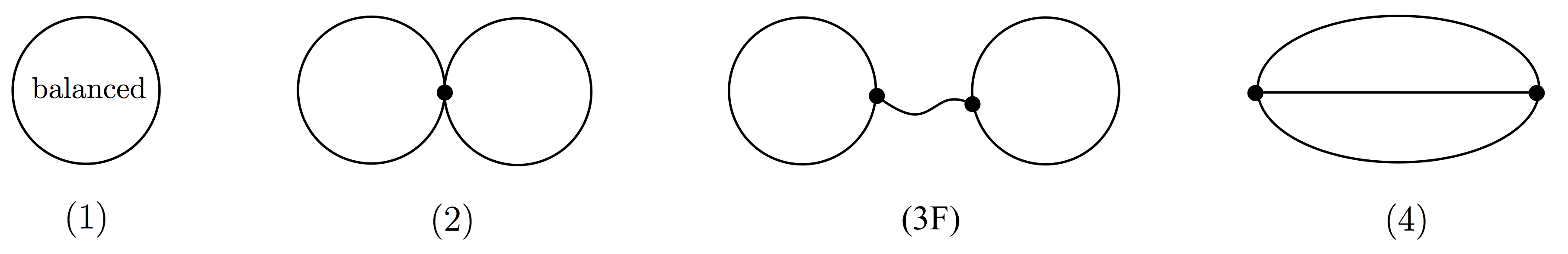Circuits of frame matroids are subdivisions of these graphs. Unlabelled cycles are unbalanced.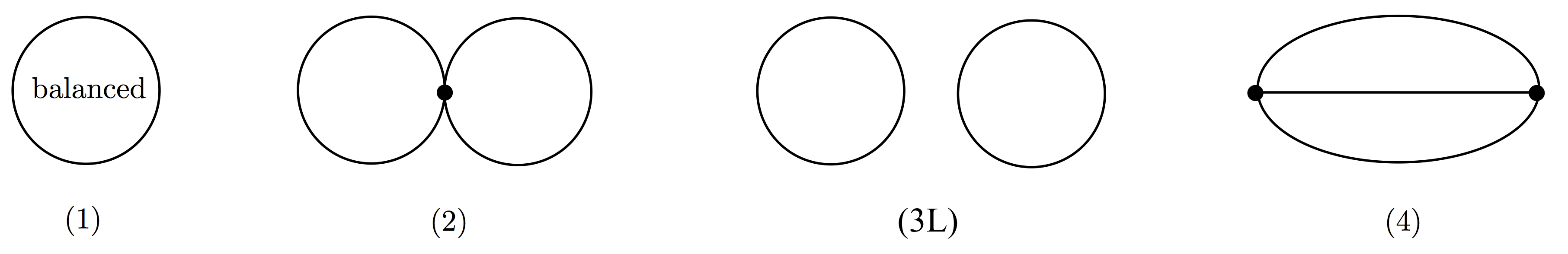Circuits of lift matroids are subdivisions of these graphs. Unlabelled cycles are unbalanced.

Let us call a subdivision of one of these five biased subgraphs (1), (2), (3F), (3L), (4), a circuit-subgraph. Note that the frame and lift matroids associated with a given biased graph differ on just one pair of these circuit-subgraphs, namely, (3F) and (3L) — a pair of vertex disjoint unbalanced cycles forms a circuit of the lift matroid, but is independent in the frame matroid. As Irene has explained in her previous posts, given a biased graph, we get a frame matroid by taking as circuits just circuit-subgraphs of the forms (1), (2), (3F), and (4), we get a lift matroid by taking as circuits just circuit-subgraphs of the forms (1), (2), (3L), and (4), and Tom Zaslavsky has shown that in fact all frame matroids, and all lift matroids, are obtained this way.

What about quasi-graphic matroids? Here is an example. The Vámos matroid (shown below left as a cube, in which the only 4-circuits are the 4 “sides” and just the one “diagonal” plane 2468) is neither a frame matroid, nor a lift matroid, but it is quasi-graphic, with the graph below right providing a framework. (Check that it satisfies the definition!)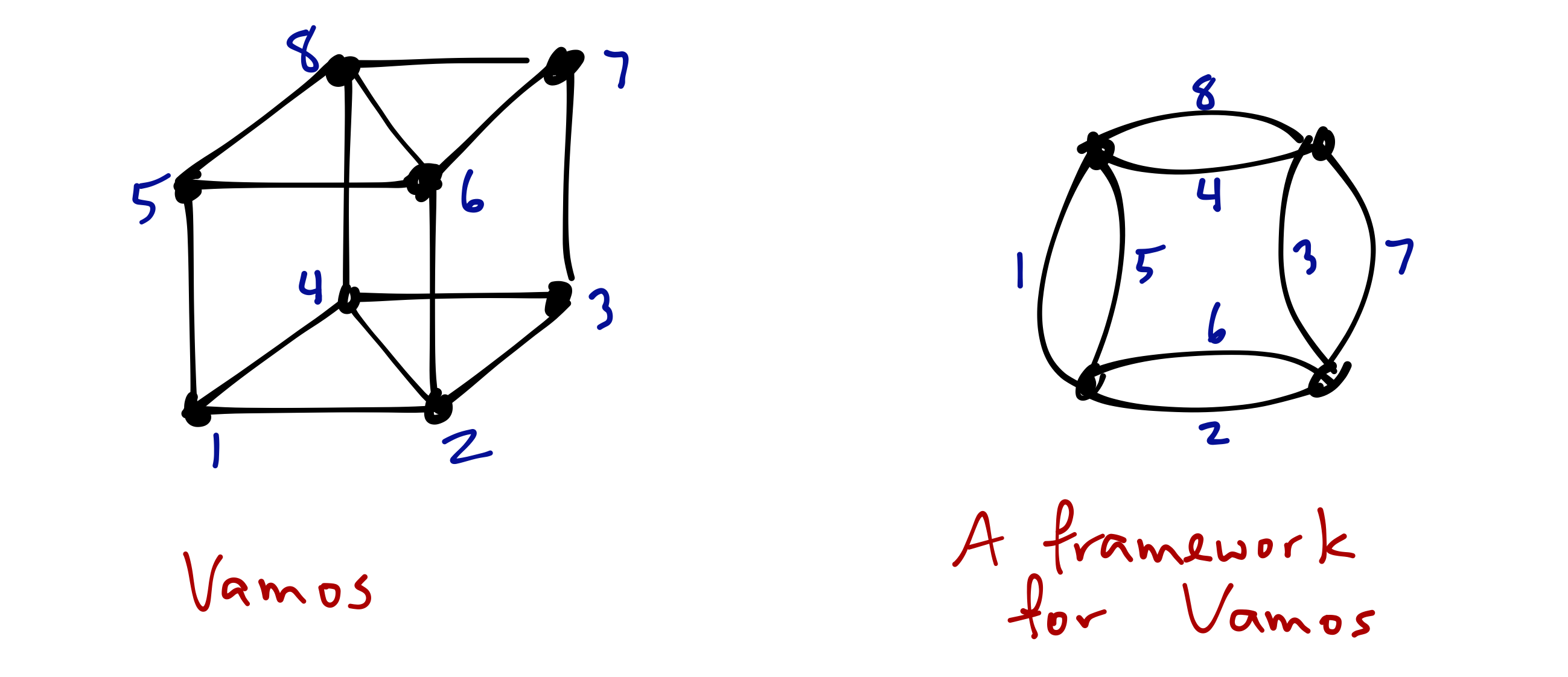Consider the 4-circuits of Vámos, and the subgraphs they form in the framework graph.
The four planes given by the front, back, and sides of the cube each form a circuit-subgraph of type (2), which is a circuit-subgraph for both frame and lift matroids. But together the circuit 2468 and the independent set 1357 prevent this graph from being either a frame or a lift representation for Vámos: circuit 2468 appears as a type (3L) circuit-subgraph, but so does the independent set 1357.

It turns out that (here I’m summarising results of [GGW17]), just as with biased graph representations of frame and lift matroids, the edge set of every cycle in a framework $G$ for a quasi-graphic matroid $M$ is either a circuit of $M$ or is independent in $M$. Further, declaring each cycle of $G$ to be balanced or unbalanced accordingly, just as for frame and lift matroids, yields a biased graph $(G,\mathcal B)$, where $\mathcal B$ denotes the collection of balanced cycles of $G$ (that is, the collection of balanced cycles satisfies the theta property: no theta subgraph contains exactly two balanced cycles). Moreover, every circuit of $M$ appears in $G$ as one of our five circuit-subgraphs (1), (2), (3F), (3L), (4). Conversely, the edge set of each circuit-subgraph of $G$ of one of the forms (1), (2), or (4) is a circuit of $M$, and each of the form of (3F) is either a circuit or contains a circuit of the form (3L).

Thus frameworks for matroids behave very much like biased graph representations for frame and lift matroids. Given a biased graph, taking $\{(1),(2),(3$F$),(4)\}$ as our circuit-subgraphs gives us a frame matroid, taking $\{(1),(2),(3$L$),(4)\}$ as our circuit-subgraphs gives us a lift matroid; and allowing all of $\{(1), (2), (3$F$), (3$L$), (4)\}$ as circuit-subgraphs gives the class of quasi-graphic matroids. This phenomenon is illustrated in the framework for the Vámos matroid above: 2468 is a (3L) circuit-subgraph, while each of the four circuits $1357 \cup e$ for $e \in \{2,4,6,8\}$ are (3F) circuit-subgraphs. Put another way, if a matroid $M$ has a framework having no circuit-subgraphs of type (3F), then we have a biased graph representation for $M$ as a lift matroid; if $M$ has a framework with no circuit-subgraphs of type (3L), then we have a biased graph representation for $M$ as a frame matroid; the Vámos matroid shows that (3F) and (3L) type circuit-subgraphs can coexist in a framework.

# Recognition of frame, and of lift matroids, is intractable

As mentioned above, Rong and Geoff have shown that there can be no algorithm that can determine, via a rank oracle, in time polynomial in the size of the ground set, whether or not a given matroid is a frame matroid. They also show no such algorithm can exist for recognising lift matroids. They do so using two particular families of quasi-graphic matroids, one for frame and one for lift, arising from the same infinite family of framework graphs. More precisely, they prove the following two theorems.

Theorem 2 [CW16]. For any polynomial $p(\cdot)$, there is a frame matroid $M$ such that, for any collection $\mathcal A$ of subsets of $E(M)$ with $|\mathcal A| \leq p(|E(M)|)$, there is a quasi-graphic matroid $N$ that is not frame, such that $E(N)=E(M)$ and for each $A \in \mathcal A$, $r_k(A) = r_M(A)$.

Theorem 3 [CW16]. For any polynomial $p(\cdot)$, there is a lift matroid $M$ such that, for any collection $\mathcal A$ of subsets of $E(M)$ with $|\mathcal A| \leq p(|E(M)|)$, there is a quasi-graphic matroid $N$ that is not lift, such that $E(N)=E(M)$ and for each $A \in \mathcal A$, $r_k(A) = r_M(A)$.

The proofs go like this. Construct an infinite family of biased graphs $(W_k, \emptyset)$. Relaxation of a particular circuit-hyperplane of the lift matroid $LM(W_k,\emptyset)$ yields a quasi-graphic matroid that is no longer lift, but which agrees with $LM(W_k,\emptyset)$ on almost all subsets. Performing the reverse tweak to the frame matroid $FM(W_k, \emptyset)$ yields a quasi-graphic matroid that is no longer frame, but which agrees with $FM(W_k,\emptyset)$ on almost all subsets. The operation reverse to relaxation of a circuit-hyperplane is that of tightening a free basis. A free basis of a matroid is a basis $B$ such that $B \cup e$ is a circuit for each $e \in E(M)-B$. If $B$ is a free basis of $M$, then removing $B$ from the set of bases of $M$ results in a matroid, which we say is obtained by tightening $B$.

Here is the family of biased graphs. For each even integer $k \geq 4$, let $W_k$ be the graph consisting of 4 edge-disjoint $k$-cycles on vertices $u_1, \ldots, u_k, v_1, \ldots, v_k$: one cycle on the $u_i$’s, one cycle on the $v_i$’s (the vertex disjoint pair of blue cycles in the drawing of $W_6$ at below left), and two cycles (red and green in figure) alternating between $u_i$’s and $v_i$’s hitting every second vertex of the blue cycles as shown (below left).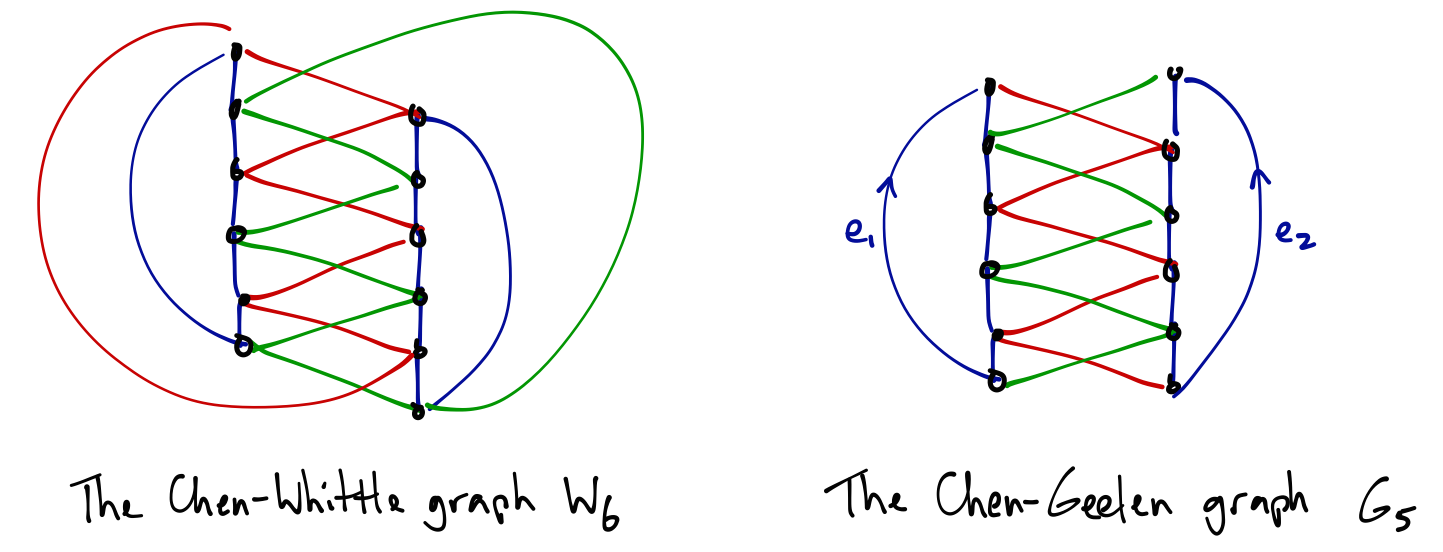Call a green edge and a red edge that cross in this drawing a crossing pair. Observe that $W_k$ has $k$ crossing pairs, and that for every collection $S$ of an even number of crossing pairs, there is a pair of disjoint cycles $C_S^1, C_S^2$ which use precisely these crossing pairs, each of which has length $k$, and which between them traverse all vertices of $W_k$. (See figure below: choosing the 2nd and 4th crossing pair defines the pair of cycles highlighted green and yellow.)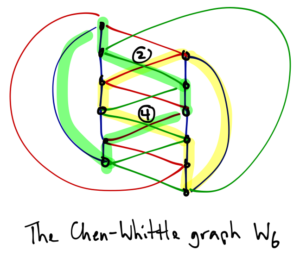The graph $W_k$ has exponentially many collections of crossing pairs: there are $2^{k-1}$ collections consisting of an even number of crossing pairs. Hence $W_k$ has $2^{k-1}$ pairs of disjoint cycles, each pair having the property that together they contain all vertices of $W_k$. Each such pair of cycles is a circuit-hyperplane of the lift matroid $LM(W_k,\emptyset)$, and a free basis of the frame matroid $FM(W_k,\emptyset)$. Let $Z$ be the edge set of a pair of cycles obtained as above from a chosen set of crossing pairs of even cardinality. Let $M_L^Z$ be the matroid obtained from $LM(W_k,\emptyset)$ by relaxing the circuit-hyperplane $Z$. Let $M_F^Z$ be the matroid obtained from $FM(W_k,\emptyset)$ by tightening the free basis $Z$. To distinguish $LM(W_k,\emptyset)$ from $M_L^Z$, and to distinguish $FM(W_k,\emptyset)$ from $M_F^Z$, requires checking the rank of $2^{k-1}$ subsets. This, of course, will be greater than any polynomial in $|E(W_k)|$ for sufficiently large $k$. Since $W_k$ remains a framework for both $M_L^Z$ and $M_F^Z$, both these matroids are quasi-graphic. The proofs are completed by showing that $M_L^Z$ is not a lift matroid, and that $M_F^Z$ is not frame (which takes just another couple of pages in Rong and Geoff’s paper).

The Chen-Whittle graph’s usefulness does not stop here.

# Excluded minors

To disprove Conjectures 1 and 2, Rong and Jim exhibit an infinite family of quasi-graphic matroids, each of which is minor-minimally not frame, and another infinite family of quasi-graphic matroids, each of which is minor-minimally not lifted-graphic. As in Rong and Geoff’s proofs of Theorems 2 and 3, these two families share a common framework. In fact, they again use the Chen-Whittle graphs!

Here is Rong and Jim’s construction. For each odd positive integer $k \geq 5$, let $G_k$ be the graph obtained from the Chen-Whittle graph $W_{k+1}$ defined above by deleting exactly one crossing pair (at right in figure above is shown the Chen-Geelen graph $G_5$). It is convenient to describe the collection of balanced cycles of $G_k$ by group-labelling (group-labelled graphs are described in Irene’s first post on biased graphs, Section 1, 4th bullet point).

For each odd positive integer $k \geq 5$, we obtain a quasi-graphic excluded minor for the class of frame matroids, with framework $G_k$, as follows. Label $G_k$ using the multiplicative group of $\mathbb R$. Referring to the drawing of $G_5$ above: orient each of $e_1$ and $e_2$ “up” from the bottom vertex to the top vertex of the vertical path making up its “side” of the crossing ladder, and assign to both $e_1$ and $e_2$ the label 2. Orient all remaining edges arbitrarily, and assign each label 1. Let $\mathcal B_k$ be the collection of balanced cycles defined by this labelling (that is, ${\mathcal B}_k$ consists of those cycles $C$ for which the product of edge labels, taken while traversing $C$ in a cyclic order, is 1, where we take the multiplicative inverse of each label whose edge is traversed against its orientation). Let $P$ be the paths forming the two vertical sides of the ladder, so $P \cup \{e_1, e_2\}$ is the pair of blue disjoint cycles in the figure, and let $Q$ be the union of the red and green paths. Then $P \cup \{e_1, e_2\}$ and $Q \cup \{e_1, e_2\}$ are free bases of $FM(G_k, \mathcal B_k)$. Let $M_k^F$ be the matroid obtained from $FM(G_k, \mathcal B_k)$ by tightening $P \cup \{e_1, e_2\}$ and $Q \cup \{e_1, e_2\}$. Then $M_k^F/\{e_1, e_2\}$, while quasi-graphic (with framework $G_k/\{e_1,e_2\}$), is an excluded minor for the class of frame matroids.

An excluded minor for the class of lift matroids is obtained in a similar manner. Again orient $e_1$ and $e_2$ up from the bottom to the top vertex of the vertical paths of the ladder. This time, label $G_k$ using elements of the additive group of integers, labelling $e_1$ with $1$, $e_2$ by $-1$, and all remaining edges with $0$. Let $\mathcal B_k$ be the collection of balanced cycles defined by this labelling (that is, $C \in \mathcal B_k$ if and only if when traversing $C$ in a chosen cyclic direction, taking the sum of the labels on its edges, subtracting the label on each edge traversed against its orientation, yields $0$). Let $P$ and $Q$ be as before. Then $P \cup \{e_1,e_2\}$ and $Q \cup \{e_1, e_2\}$ are circuit-hyperplanes of $LM(G_k, \mathcal B_k)$. Let $M_k^L$ be the matroid obtained from $LM(G_k, \mathcal B_k)$ by relaxing $P \cup \{e_1, e_2\}$ and $Q \cup \{e_1, e_2\}$. Then $M_k^L / \{e_1, e_2\}$, while quasi-graphic, is an excluded minor for the class of lift matroids.

Rong and Jim make the following conjecture.

Conjecture 3 [CG17]. The class of quasi-graphic matroids has only a finite number of excluded minors.

Dillon Mayhew and I have recently proved the following three theorems.

Theorem 4 [FM17]. The class of frame matroids has only a finite number of excluded minors of any fixed rank.

Theorem 5 [FM17]. The class of lift matroids has only a finite number of excluded minors of any fixed rank.

Theorem 6 [FM17]. The class of quasi-graphic matroids has only a finite number of excluded minors of any fixed rank.

Rong and Jim’s counterexamples to Conjecture 1 and 2 are both infinite families of excluded minors of ever increasing, arbitrarily large rank (if $G$ is a connected framework for a non-graphic quasi-graphic matroid $M$, then the rank of $M$ is $|V(G)|$). Theorems 4 and 5 say that any such collections of excluded minors for these classes must have this property. Theorem 6 provides evidence toward Conjecture 3 — though no more evidence than Theorems 4 and 5, respectively, provide toward Conjectures 1 and 2! What is perhaps interesting about Theorems 4, 5, and 6 is that — in contrast to what we’ve just seen in the results of Rong, Geoff, and Jim above — the same strategy works for all three classes. Dillon and I set out to prove Theorem 4, and having done so, realised that essentially the same proof also gives us Theorems 5 and 6. Perhaps we can take a look at this strategy in a subsequent post.

# References

[CG17] Rong Chen and Jim Geelen. Infinitly many excluded minors for frame matroids and for lifted-graphic matroids. arXiv:1703.04857

[CW16] Rong Chen and Geoff Whittle. On recognising frame and lifted-graphic matroids. arXiv:1601.01791

[FM17] Daryl Funk and Dillon Mayhew. On excluded minors for classes of graphical matroids. Forthcoming.

[GGW17] Jim Geelen, Bert Gerards, and Geoff Whittle. Quasi-graphic matroids. arXiv:1512.03005

[S81] Paul Seymour. Recognizing graphic matroids. Combinatorica (1981). MR602418

# A Taste of Tangles

Guest post by Tara Fife

This semester, I’ve been doing a reading course with Stefan van Zwam, the bulk of which involved reading interesting papers about tangles. This post highlights some of my favorite ideas so far. We start with an example that essentially comes from Reinhard Diestel and Geoff Whittle’s paper “Tangles and the Mona Lisa” . The goal is to illustrate the intuition behind some of the definitions related to tangles. Precise definitions for connectivity systems and tangles as related to pictures can be found in . Here is a picture of my brother Daniel and me on a ferry outside Seattle.If this picture were printed out and cut into two pieces, then we could sometimes decide that one piece was less important than the other. For instance, if we cut along the red lines below, it is clear that the center piece, while missing some of the beautiful scenery, is the important part of the picture from the perspective of capturing the human subjects of the picture.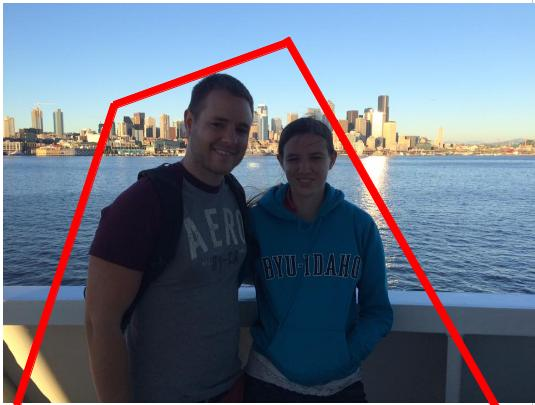If, however, we cut the picture with a squiggly cut, as below, then perhaps neither piece is unimportant. A connectivity system $K=(E(K),\lambda_K)$ consists of a finite set, $E(K)$, together with a connectivity function $\lambda _K:2^{E(K)}\rightarrow\mathbb{R}$ that gives us a way to rank the size of cuts. As such, we expect $\lambda_K(X)=\lambda_K(E(K)-X)$, for all subsets $X$ of $E(K)$. That is, we expect $\lambda_K$ to be symmetric. We also want our connectivity function to be submodular, that is, $\lambda_K(X)+\lambda_K(Y)\geq \lambda_K(X\cap Y)+\lambda_K(X\cup Y)$ for all subsets $X$ and $Y$ of $E(K)$. Any function which is symmetric and submodular is a connectivity function.If $\lambda_1$ and $\lambda_2$ are connectivity functions on the same set, then it is straightforward to check that so is $\lambda_1+\lambda_2$ and, for a positive constant $c$, both $c\cdot\lambda_1$and $\lambda_1+c$. If $K=(E,\lambda_K)$ and $K’=(E,\lambda_{K’})$ are connectivity systems, then we say that $K’$ is a tie breaker if $\lambda_{K’}(X)\leq \lambda_{K’}(Y)$ whenever $\lambda_K(X)\leq \lambda_K(Y)$ and $\lambda_{K’}(X)\not=\lambda_{K’}(Y)$ unless $X=Y$ or $X=E-Y$. Geelen, Gerards, and Whittle’s “Tangles, tree-decompositions and grids in matroids”  proves the following.

Lemma 1 All connectivity functions have tie breakers.

Proof. Let $K=(E,\lambda)$ be a connectivity function. We can assume that $E=\{1, 2, \ldots, n\}$ Define $\lambda_L$ by $\lambda_L(X)=\sum_{i\in X}2^i$ for $X\subseteq {1, 2,\ldots, n-1}$, and $\lambda(X)=\lambda(E-X)$ for $X$ containing $n$. We need to show that $\lambda_L$ is submodular. If $X,Y\subseteq\{1, 2,\ldots, n-1\}$, then
\begin{align*}
\lambda(X)+\lambda(Y) & =\sum_{i\in X}2^i+\sum_{i\in Y}2^i=\sum_{i\in X\cap Y}2^i+\sum_{i\in X-Y}2^i+\sum_{i\in Y}2^i=\sum_{i\in X\cap Y}2^i+\sum_{i\in X\cup Y}2^i\\
& =\lambda_L(X\cap Y)+\lambda_L(X\cup Y).
\end{align*}

If $X\subseteq\{1,2,\ldots,n-1\}$ and $n\in Y$, then
\begin{align*}
\lambda(X)+\lambda(Y) & =\sum_{i\in X}2^i+\sum_{i\not\in Y}2^i=\sum_{i\in X\cap Y}2^i+\sum_{i\in X-Y}2^i+\sum_{i\not\in Y\cup X}2^i+\sum_{i\not\in X-Y}2^i\\
& \geq\sum_{i\in X\cap Y}2^i+\sum_{i\not\in X\cap Y}2^i=\lambda_L(X\cap Y)+\lambda_L(X\cup Y).
\end{align*}

If $n\in X$ and $n\in Y$, then
\begin{align*}
\lambda_L(X)+\lambda_L(Y) & =\lambda_L(E-X)+\lambda_L(E-Y)\\
& =\lambda_L((E-X)\cap(E-Y))+\lambda_L((E-X)\cup(E-Y))\\
& =\lambda_L(E-(X\cup Y))+\lambda_L(E-(X\cap Y)).
\end{align*}

Thus $\lambda_L$ is a connectivity function. Now let $\lambda'(X)=2^n\cdot\lambda(X)+\lambda_L(X)$. It is straightforward to check that $K’=(E,\lambda’)$ is a tie breaker for $K$.

$\square$

A tangle can be thought of as a collection $\mathcal{T}$ of less important (or small ) pieces. So far, we expect that the tangle should only make decisions about relatively simple cuts, and that the tangle should make a decision for all of the simple cuts. If we decide that the two pieces cut out of the picture below are both small, then we don’t want the part of the picture that is left to be contained in a small piece. More precisely, if $K$ is a connectivity system, then a collection $\mathcal{T}$ is a tangle of order $\theta$ in $K$ if

(T1)
$\lambda_K(A) < \theta$, for all $A \in \mathcal{T}$.
(T2)
For each separation $(A,B)$ of order less that $\theta$, either $A\in\mathcal{T}$ or $B\in\mathcal{T}$.
(T3)
If $A,B,C\in\mathcal{T}$, then $A\cup B\cup C \not=E(K)$.
(T4)
$E(K)-e$ is not in $\mathcal{T}$, for each $e\in E(K)$.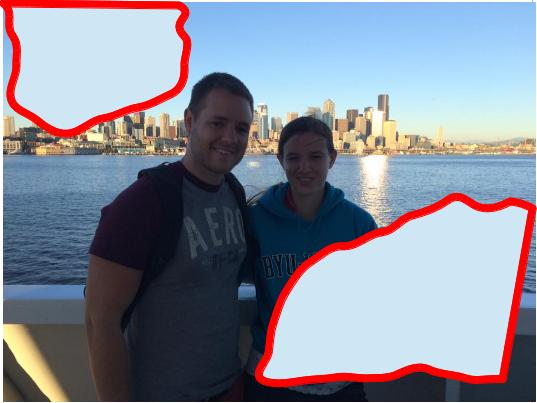For some types of cuts, there might be different opinions about which part is less important. For instance, in the following picture, the matroid community would probably say that the part containing my brother was slightly less important, because the other half contains someone who at least knows the definition of a matroid, while my brother’s company would probably say that the part that contains me is slightly less important.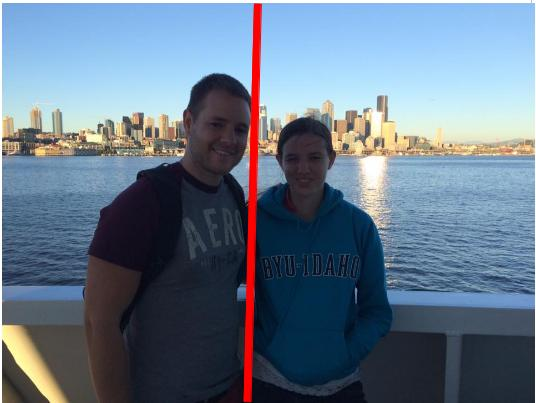My mother would not consider either part unimportant, as we are both somewhat meaningful to her. Since the tangle induced by the opinion of the matroid community disagrees with the one induced by the opinion of Daniel’s company about which half of the separation above is less important, that separation is called a distinguishing separation. Since the tangle induced by my mother’s opinions (that is, a piece is only unimportant if it avoids me and avoids Daniel) is a subset of the matroid community tangle (where a piece is unimportant if it avoids me) and a subset of the company tangle (where a piece in unimportant if it avoids Daniel), it is called a truncation of either of them. That is, $\mathcal{T}’$ is a truncation of $\mathcal{T}$ if $\mathcal{T}’\subseteq\mathcal{T}$. If a tangle is not a proper truncation of another tangle, then it is a maximal tangle.

The above definition of a connectivity function includes the usual connectivity function of a matroid $M$, namely, $\lambda(X)=r(X)+r(E-X)-r(M)$. One of the main results of  gives us information about maximal tangles in a matroid. Before we state the theorem, we need to introduce a bit more notation. If $K=(E,\lambda)$ is a connectivity system, $T$ is a tree, and $\mathcal{P}$ a function from $E$ to $V(T)$, then we say that $(T,\mathcal{P})$ is a tree decomposition of $E$. For a subset $X$ of $V(T)$, we define $\mathcal{P}(X)=\{e:P(e)\in X\}$, and for a subtree $T’$ of $T$, we define $\mathcal{P}(T’)=\mathcal{P}(V(T’))$. We say that a separation $(A,B)$ of $K$ is displayed by $(T,\mathcal{P})$ if $A=\mathcal{P}(T’)$ for some subtree $T’$ of $V(T)$ obtained by deleting an edge of $T$ and letting $T’$ be one of the resulting connected components.The order if a separation $(A,B)$ is $\lambda(A)=\lambda(B)$.

Theorem 1

Let $K$ be a connectivity system, and let $\mathcal{T}_1,\mathcal{T}_2,\ldots,\mathcal{T}_n$ be maximal tangles in $K$. Then there is a tree decomposition $(T,\mathcal{P})$ of $E(K)$ with $V(T)=[n]$ such that the following hold.

1. For each $i\in V(T)$ and $e\in E(T)$, if $T’$ is the component of $T-e$ containing $i$, then $\mathcal{P}(T’)$ is not in $\mathcal{T}_i$.
2. For each pair of distinct vertices $i$ and $j$ of $T$, there is a minimum-order distinguishing separation for $\mathcal{T}_i$ and $\mathcal{T}_j$ displayed by $T$.

Before we sketch a proof of this theorem, we give an example that illustrates the statement of the theorem.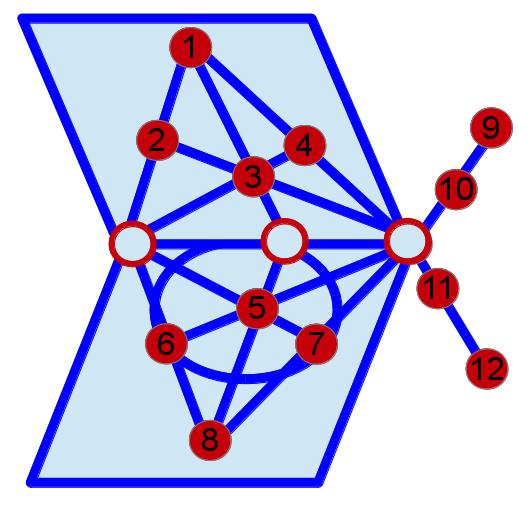Consider the matroid, $M=(,\mathcal{I})$ illustrated above. We first need to determine what the maximal tangles are. Let $S_1=\{9,10\}$, $S_2=\{11,12\}$, and $S_3=$. We will show that an order-1 tangle of $M$ has the form the union of $\{S_i,S_j,(S_i\cup S_j)\}$ together with the set of singletons, where $i$ and $j$ are distinct members of $\{1,2,3\}$.

Let $\mathcal{T}$ be an order-1 tangle of $M$. The sets with connectivity 1 consist of the 12 singletons, their complements, and $S_1$, $S_2$, $S_3$, and their complements. By (T3), at most two of $S_1, S_2, S_3$ are in $\mathcal{T}$. Assume that $\{i,j,k\}=\{1,2,3\}$, and that $S_k$ is not in $\mathcal{T}$. Since $(S_i\cup S_j,S_k)$ is an order-1 separation of $M$, by (T2) $S_i\cup S_j$ is in $\mathcal{T}$. By (T3) (and the fact that there are at least 3 elements of $\mathcal{T}$), we get that neither $S_j\cup S_k$ nor $S_i\cup S_k$ is in $\mathcal{T}$, so by (T2), $S_i$ and $S_j$ are in $\mathcal{T}$.
Since singletons must all be in every tangle of order at least 1, the result follows.

The sets with connectivity 2 either contain $\{1,2,3,4\}$ and avoid $\{5,6,7,8\}$ or contain $\{5,6,7,8\}$ and avoid $\{1,2,3,4\}$. Arguing as above, we get that that the order-2 tangles of $M$ are $\{X:\lambda(X)\leq 2 \text{ and } S\not\subseteq X\}$ for $S\in\{\{1,2,3,4\}, \{5,6,7,8\}\}$. We note that the order-1 tangle where $\{S_i,S_j\}=\{\{1,2,3,4\},\{5,6,7,8\}\}$ is a truncation of both of the order-2 tangles, and that the other order-1 tangles can be represented as $\{X:\lambda(X)\leq 1 \text{ and } \{1,2, 3, 4\}\not\subseteq X\}$ and $\{X:\lambda(X)\leq 1 \text{ and } \{5,6, 7, 8\}\not\subseteq X\}$. Since there are no separations of order-3 or more in $M$, the four maximal tangles of $M$ are $\mathcal{T}_S=\{X:\lambda(X)\leq \lambda(S) \text{ and } S\not\subseteq X\}$, for $S\in\{\{1,2,3,4\},\{5,6,7,8\},\{9,10\},\{11,12\}\}$. For $S \in \{\{1,2,3,4\}, \{9,10\},\{11,12\}\}$, a minimum-order distinguishing separation for $\mathcal{T}_S$ and $\mathcal{T}_{S’}$ is $(S,-S)$, which is displayed by the tree, $T$, below.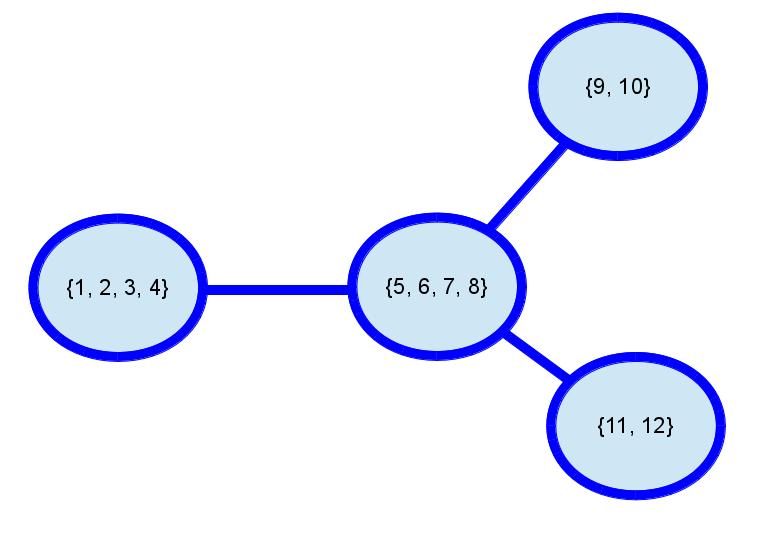The above argument showing that if $S_i\cup S_j$ is in $\mathcal{T}$, then each of $S_i$ and $S_j$ are in $\mathcal{T}$, can be generalized to show the following.

Lemma 2

If $A$ is in a tangle, $\mathcal{T}$ of $K$ of order $\theta$, and $B\subseteq A$ with $\lambda_K(B)\leq\theta$, then $B\in\mathcal{T}$.

To prove Theorem 1, we actually prove the following slightly stronger result also from .

Theorem 2

Let $K$ be a connectivity system, and let $\mathcal{T}_1,\mathcal{T}_2,\ldots,\mathcal{T}_n$ be tangles in $K$, none a truncation of another. Then there is a tree decomposition $(T,\mathcal{P})$ of $E(K)$ with $V(T)=[n]$ such that the following hold.

1. For each $i\in V(T)$ and $e\in E(T)$, if $T’$ is the component of $T-e$ containing $i$, then $\mathcal{P}(T’)$ is not in $\mathcal{T}_i$.
2. For each pair of distinct vertices $i$ and $j$ of $T$, there is a minimum-order distinguishing separation for $\mathcal{T}_i$ and $\mathcal{T}_j$ displayed by $T$.

Proof Sketch
If $K$ is a connectivity system and $K’$ is a tie breaker for $K$, then it is easy to see that a tangle $\mathcal{T}$ of $K$ is also a tangle of $K’$, so it is safe to assume that $K$ is its own tie breaker.

Since $K$ is its own tie breaker, for each distinct $i$ and $j$ in $[n]$, there is a minimum-order separation $(X_{ij},Y_{ij})$ of $K$ distinguishing $\mathcal{T}_i$ and $\mathcal{T}_j$, where $X_{ij}\in\mathcal{T}_i$. Using some well known results (whose statements require terminology which we haven’t introduced), it can be show that there is a tree decomposition $(T,\mathcal{P})$ of $E(K)$ such that each separation $(X_{ij},Y_{ij})$ is displayed by $(T,\mathcal{P})$, that these are the only separations displayed by $(T,\mathcal{P})$, and that each edge of $T$ displays a proper and distinct separation. It remains to show that there is a bijection from $\{\mathcal{T}_1,\ldots,\mathcal{T}_n\}$ to the vertices of $T$ satisfying the conclusion of Theorem 2.

For $i\in[n]$, let $\chi_i$ be the set of subsets $X$ of $V(T)$ such that $E(K)-\mathcal{P}[X]\in\mathcal{T}_i$ and such that $(E(K)-\mathcal{P}[X],\mathcal{P}[X])$ is displayed by $(T,\mathcal{P})$. Each member of $\chi_i$ induces a sub-tree of $T$, and, by (T3), each two members of $\chi_i$ intersect, so the intersection of the members of $\chi_i$ is non-empty. Call this intersection $V_i$. Each edge of $T$ that leaves $V_i$ displays a separation $(A,B)$ with $\mathcal{P}[V_i]\subseteq A$ and $B\in\mathcal{T}_i$. The proof concludes by showing that the $V_i$’s partition the vertices of $T$ into singletons, since this shows that $|V(T)|=n$, and since vertices in $V_i$ satisfy the condition (1) of the theorem.

For each $i\not=j$, the set $\mathcal(V_i)$ is in $Y_{ij}$ and the set $\mathcal(V_j)$ is in $Y_{ji}=X_{ij}$, so the sets $V_1, \ldots, V_n$ are disjoint.

Suppose that $w\in V_i$. We need to show that $\{w\}=V_i$. Choose the edge $e$ incident with $w$ that displays the separation $(X_{ij},Y_{ij})$ of strictly largest order. Since we are assuming that $K$ is its own tie breaker, there is a strictly largest order. Now, each of the other edges incident with $w$ displays a separation of order less than the order displayed by $e$, and hence, less than the orders of $\mathcal{T}_i$ and $\mathcal{T}_j$. Then, by the definition of $(X_{ij},Y_{ij})$, none of these separations distinguish $\mathcal{T}_i$ and $\mathcal{T}_j$. By the definition of $V_i$ and the fact that $X_{ij}$ is in $\mathcal{T}_i$, we know that $\mathcal{P}(w)$ is not a subset of $X_{ij}$ so it is a subset of $Y_{ij}$. Then by Lemma 2, for each of the separations induced by edges other than $e$ incident to $w$, the set not containing $\mathcal{P}(w)$ is in $\mathcal{T}_j$, and hence it is in $\mathcal{T_i}$. Thus, $V_i=\{w\}$.
$\square$

The complete details of the last proof can be found in  along with a corollary which bounds the number of maximal tangles.

As hinted at in the example, tangles can end up pointing to “highly” connected regions of the matroid. This is useful in structure theory because the highly connected regions usually contain the structure that we care about, and a tangle can be used to identify where deletions and contractions can be made while preserving the structure. This idea is utilized, for example, in [3, 4]. Tangles in general grew out of a definition for tangles of graphs, which were used to prove that finite graphs are well quasi ordered.

# References

 R. Diestel, and G. Whittle, Tangles and the Mona Lisa, arXiv:1603.06652v2 [math.CO]

 J. Geelen, B. Gerards, and G. Whittle, Tangles, tree-decompositions and grids in matroids, J. Combin. Theory Ser. B 99 (2009) 657-667

 J. Geelen, and S. van Zwam, Matroid 3-connectivity and branch width, arXiv:1107.3914v2 [math.CO]

 P. Nelson, and S. van Zwam, Matroids representable over fields with a common subfield, arXiv:1401.7040v1 [math.CO]

 N. Robertson and P. D. Seymour, Graph minors, X. Obstructions to tree-decomposition. J. Combin. Theory Ser. B, 52(2):153-190, 1991.